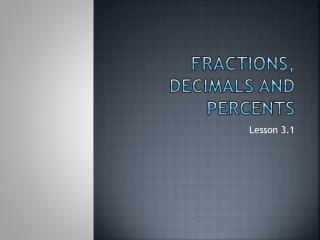DownloadDownload PresentationFractions, Decimals and Percents

# Fractions, Decimals and Percents

Télécharger la présentation## Fractions, Decimals and Percents

- - - - - - - - - - - - - - - - - - - - - - - - - - - E N D - - - - - - - - - - - - - - - - - - - - - - - - - - -
##### Presentation Transcript

1. Fractions, Decimals and Percents Lesson 3.1

2. Fractions to decimals Numbers can be written in both fraction and decimal form. For example: 3 can be written as 3/1 and 3.0 A fraction illustrates division 1/10 means 1÷10 1/10 in decimal form is .1

3. Review of decimals Each decimal place has a name. Tenths – the first number after the decimal Hundredths – the second number after the decimal Thousandths – the third number in the decimal 63.165

4. Terminating decimals • Decimals that have a definite number of decimal places are called terminating decimals. • Examples: 0.1, 0.25, 0.94 • These decimals always end.

5. Repeating decimals • When digits repeat themselves forever, they are called repeating decimals. • Examples are: 0.333333, 0.754444, 0.121212 • Repeating decimals have a bar drawn over the digits that repeat. • 4/33 = 0.121212121...can be written as 0.12

6. Patterns • Patterns can occur when we write fractions in decimal form. • Example: 1/99 = 0.01, 2/99 = 0.02, 15/99 = 0.15, 43/99 = __________ For fractions with a denominator of 99, the digits in the numerator of the fraction are the repeating digits in the decimal.

7. Finding the decimal... • If I have a fraction with a denominator of 10, the numerator is the decimal to the tenths place. • Example: 1/10 = .1 3/10 = .3 7/10 = ________ The decimal is a terminating decimal with one decimal place (the tenths place)

8. Hundreds... • If the fraction has a denominator of 100, the numerator is the decimal to the hundredths place. • Example: 26/100 = .26 72/100 = .72 39/100 = ________ If the fraction is less than 10/100, the first number after the decimal is a 0. Example: 7/100 = .07 9/100 = ____________

9. Thousands... • If the denominator is 1000, the numerator is the decimal to the thousandths place. • Example: 576/1000 = .576 112/1000 = .112 423/1000 = _________ If the number is less than 100, the first number after the decimal is a 0. Ex. 98/1000 = .098 If the number is less than 10, the first two numbers after the decimal are 0. Ex. 8/1000 = .008

10. Converting fractions to decimals... • What if I get a fraction without a 10, 100, or 1000? • First, ask yourself if the denominator can be changed to a 10, 100, or 1000. For example: 1/5 Can the denominator be changed to a 10? YES! Multiply 5 x 2. Then, what you do to bottom, you do to the top! Multiply 1 x 2. Your new fraction is 2/10... What is the decimal value?

11. Or try this... • 20/50 – Can 50 turn into 100? • YES! Multiply 50 x 2 = 100, multiply 20 x 2 = 40. New fraction = 40/100... • What is the decimal value?

12. Or how about this? • 90/200? • Multiply 200 x 5 = 1000 and 90 x 5 = 450 • New fraction = 450/1000 • What is the decimal?

13. Find equivalent fractions with denominators of 10, 100 or 1000 for the following fractions. Then, find the decimal value. • 6/20 • 18/25 • 40/50 • 60/200

14. AHH! There is no equivalent! • 9/40...I cannot turn this into a fraction with a denominator of 10, 100 or 1000...NOW WHAT?? • Remember long division?? • Yes, you need to know how.

15. 10/15 • We cannot turn 15 into 10, 100 or 1000, so, set up a long division question. • Remember, the 15 sits in the nook of the division sign and the 10 sits under the lid. • 15√10 • 15 cannot go into 10, so follow the steps of long division to find your answer.

16. Division definitions... • Divisor – a number by which a number is going to be divided. • Dividend – the amount you want to divide up. • Quotient – the answer in a division question.

17. Extra reminder... • http://www.wisc-online.com/objects/ViewObject.aspx?ID=ABM1001 • This link will show you the steps of division.

18. Try converting these fractions to decimals using long division. • 4/12 • 6/15 • 3/16

19. Homework • Workbook 3.1 • Textbook Page 88-90 #2,3,4,5,10,11,REFLECT